# C语言博客作业04--数组

| 这个作业属于哪个班级 |

| ---- | ---- | ---- |
| 这个作业的地址 |
| 这个作业的目标 | 学习数组相关内容 |
| 姓名 | 黄静 |

# 0. 展示PTA总分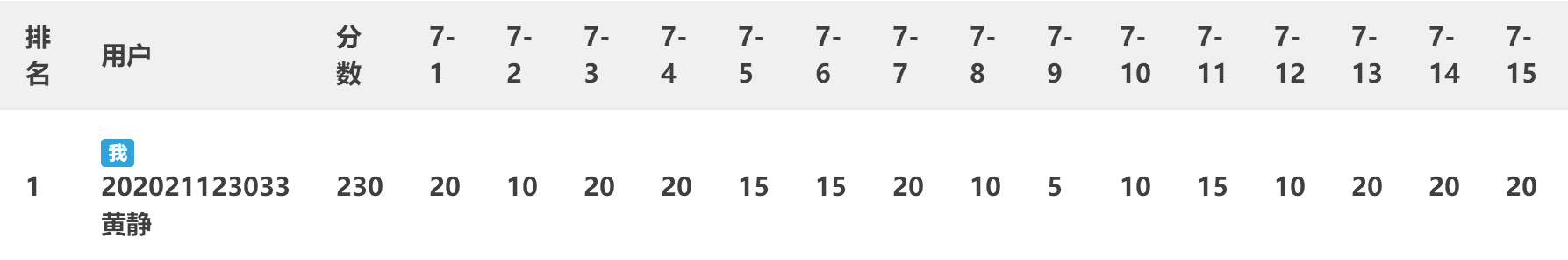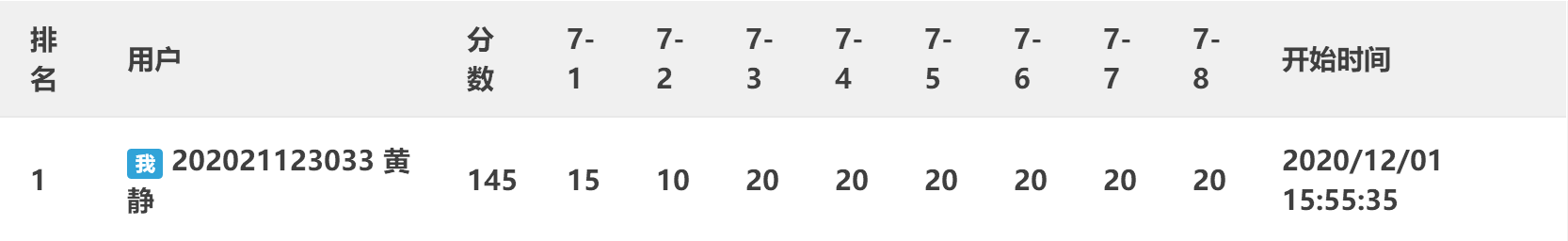# 1. 本章学习总结

## 1.1 学习内容总结

### 1.1.1 基础知识

int a;//定义一个一维数组a，并在内存中开辟了十个连续单元，用于存放数组a中的十个元素
int a;//定义一个二维数组a，存放四行四列的元素
char a;//定义一个字符数组a，实际存放九个字符，还有一个用于存放字符串结束符号'\0'


• 一维数组
for(i=0;i<n;i++)
{
scanf("%d",&a[i]);
}

• 二维数组
for(i=0;i<n;i++)
{
for(j=0;j<n;j++)
{
scanf("%d",&a[i][j]);
}
}

• 字符数组
//第一种:只能输入不含空格的字符串
scanf("%s",str)；

//第二种:循环输入，直到输入到结束标志后还得输入'\0'
while ((str[i] = getchar()) != '\n') i++;      //结束标志'\n'可为其它字符
str[i] = '\0';      //'\0'等同于0

str[i] = 0;      //  0等同于'\0'

//第三种:接受带空格字符串，输入长度少于n-1，会多带一个换行符

fgets(str,7,stbin);      //数组名+数组长度+stbin，stbin表示标准输入流



static int a;      //全初始化为0

int a={"1,2,3"}      //自定义初始化为多少，初始化几个数

static char s[]={"Happy"};
static char s[]="Happy";


• 一维数组
for(i=0;i<n;i++)
{
printf("%d",&a[i]);
}

• 二维数组
for(i=0;i<n;i++)
{
for(j=0;j<n;j++)
{
printf("%d",&a[i][j]);
}
printf("\n");
}

• 字符数组
//第一种:只能输出不含空格的字符串
printf("%s",str)；

//第二种:循环输出
for (i = 0; i < k; i++)
{
printf("%c", str[i]);
}

//第三种:接受带空格字符串

fputs(str）



字符数组中：'a'与"a"不同，"a"代表'a'和'\0' 字符数组最后必须要有结束符'\0'

### 1.1.2 查找数据

#### 1.1.2.1 顺序查找

按一定顺序将数组中各个数据与待查数据进行比较，看是否有与要查数据相等的数据

7-2 查找整数 (10分)题目详情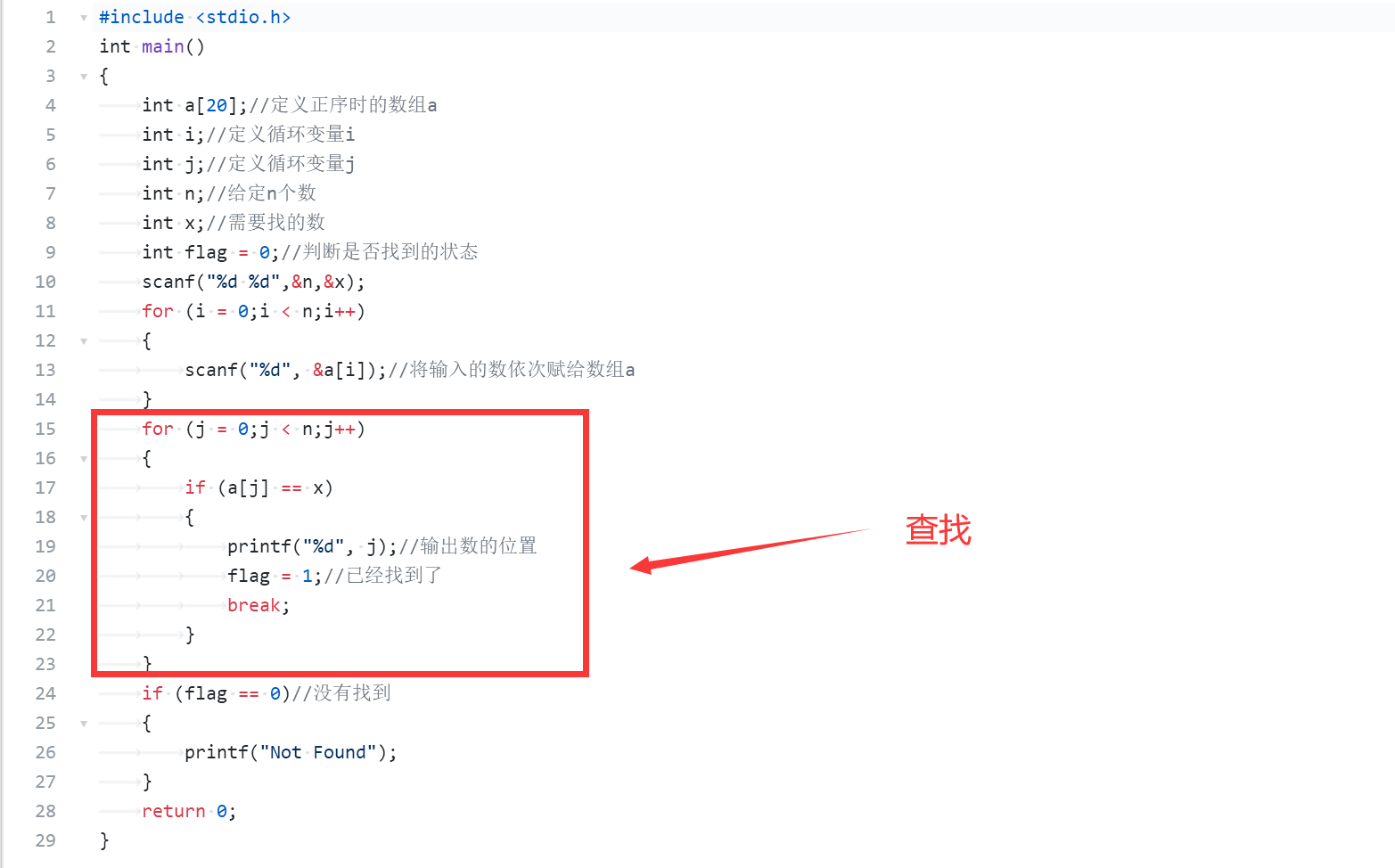#### 1.1.2.2 二分查找

定义：数组a[]，查找的数 number ，下界下标 low ，上界下标 high ，下标中间值 mid ，表示是否存在的状态 flag = 0 ；
low = 0，high = n - 1 ；
if number 比 a 小或比 a[n - 1]大  //number不在数组范围内
flag = -1 ；
end if

while (flag == 0 && low不超过high)
min = low与high之和 / 2；
if number等于a[mid]
flag = mid;
输出：是该数组中第flag + 1个数
break；
end if
else if number 小于 a[mid]  high = mid - 1；//在mid左半边找
else low = mid + 1；//在mid右半边找
end while；

if(flag==-1||low大于high)   输出：该数不在数组中；


### 1.1.3 插入数据

#### 1.1.3.1 数组右移

假设在一个从小到大排列的有序数组中：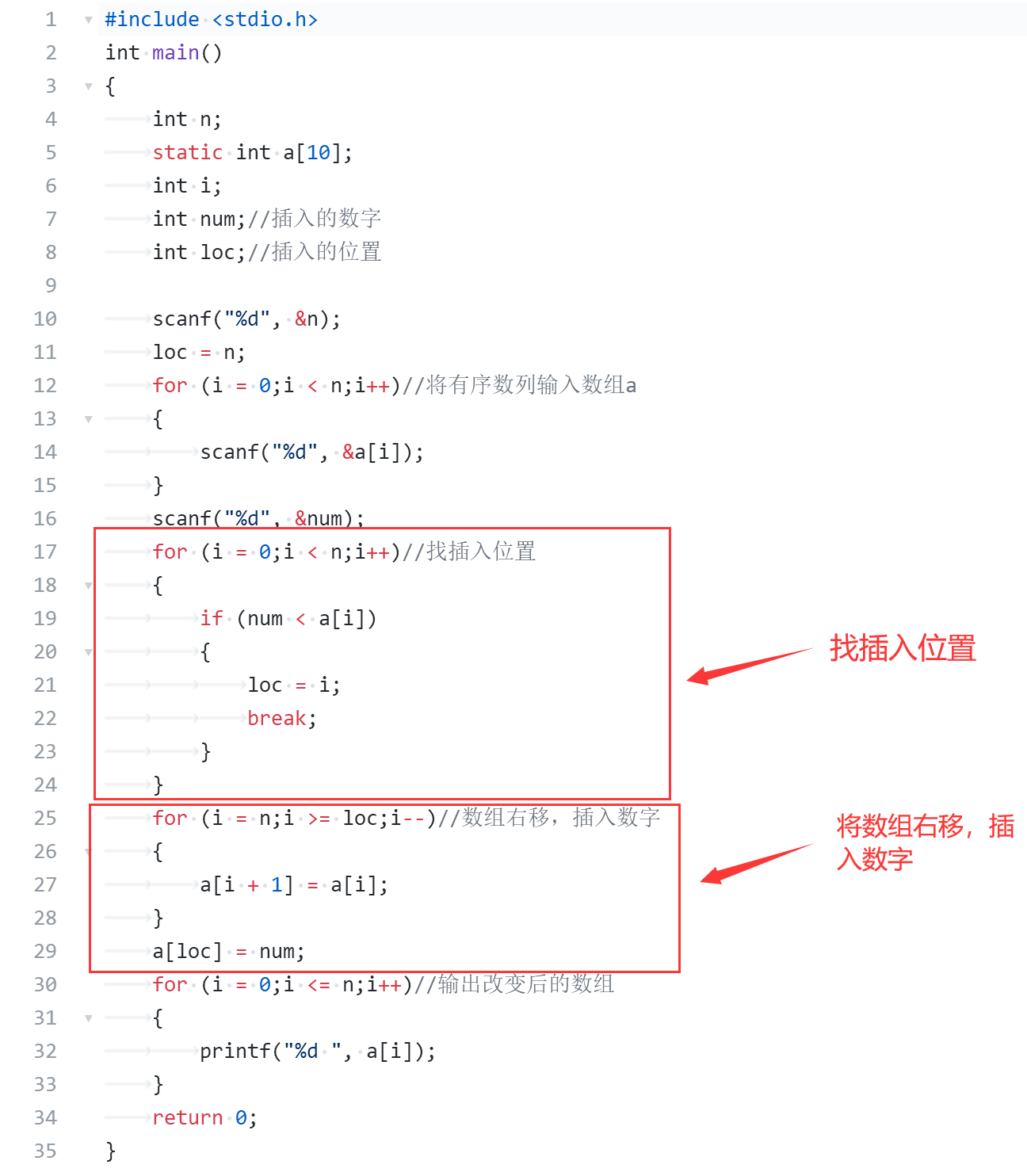### 1.1.4 数组中删除数据

#### 1.1.4.1 数组左移

遍历数组，在找到要删除的数据后，将该数据后的每个数据向左移动一位，从而达到删除此数据的目的。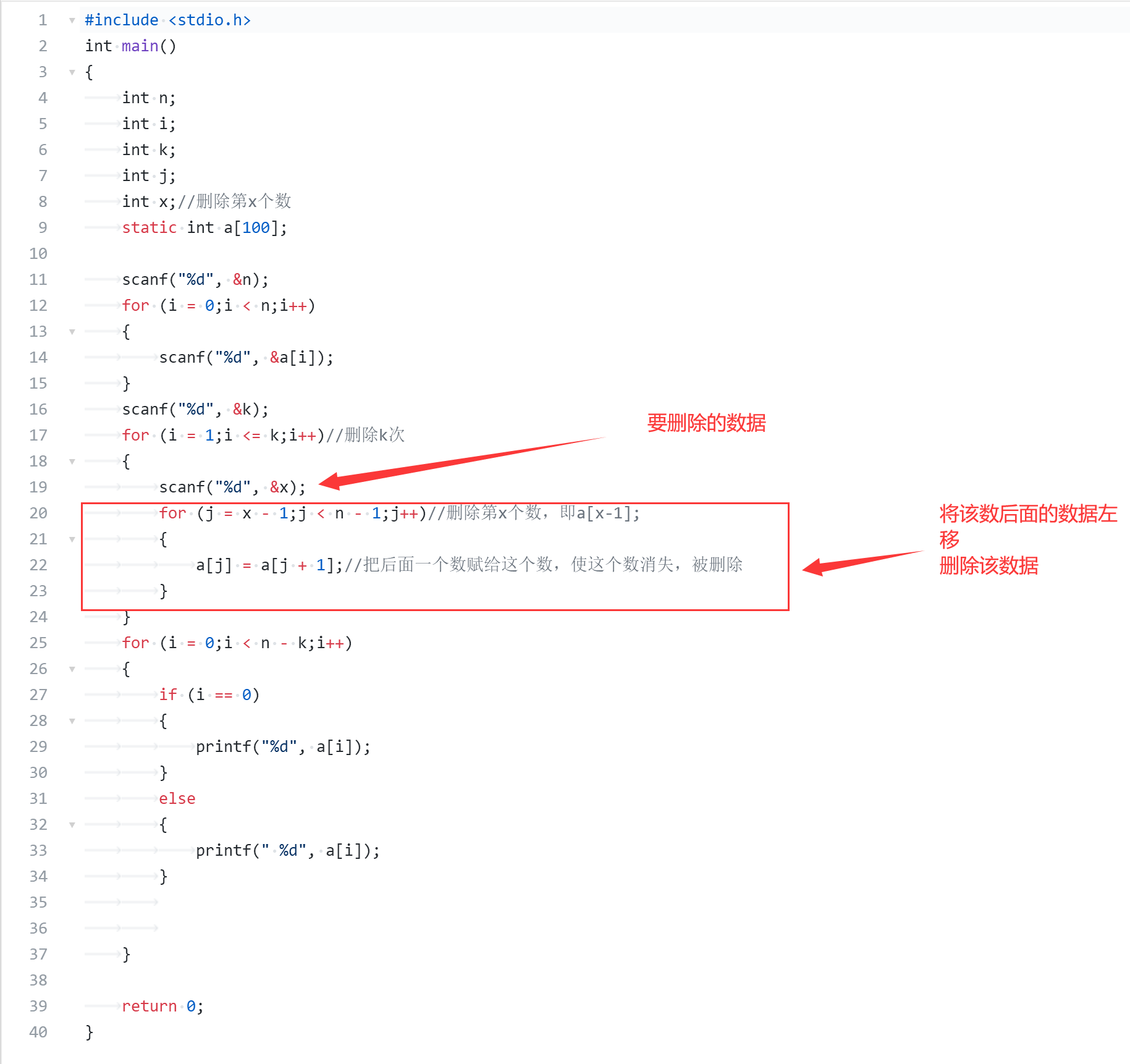#### 1.1.4.2 重构数组

在原先的数组上重新定义一个循环变量j，当数组str[i]!='\0'时，如果str[i]不等于需要被删除的数，那么重构的这个数组就等于这个数，从而使不需要被删除的数重新单独储存到以j为变量的str数组内。


定义：数组 str，循环变量 i，j；

i=j=0；
while str[i]不等于'\0'
if str[i]不等于需要被删除的数据
str[j++] 等于 str[i]；      //使用循环变量 j 将数组重构
end if
i++；
end while
str[j]等于结束符'\0' ；      //最后输入结束符,重构完成


### 1.1.5 数据排序

#### 1.1.5.1 选择排序

以从小到大排列为例：

···      ···      ···



for i=0 to n-2 do      //循环第i趟
index=i;  //记录最小下标
for j=i to n-1 do  //找最小值min对应下标
if a[index]大于a[j]
index=i;
end if
end for
交换a[index]和a[i]
end for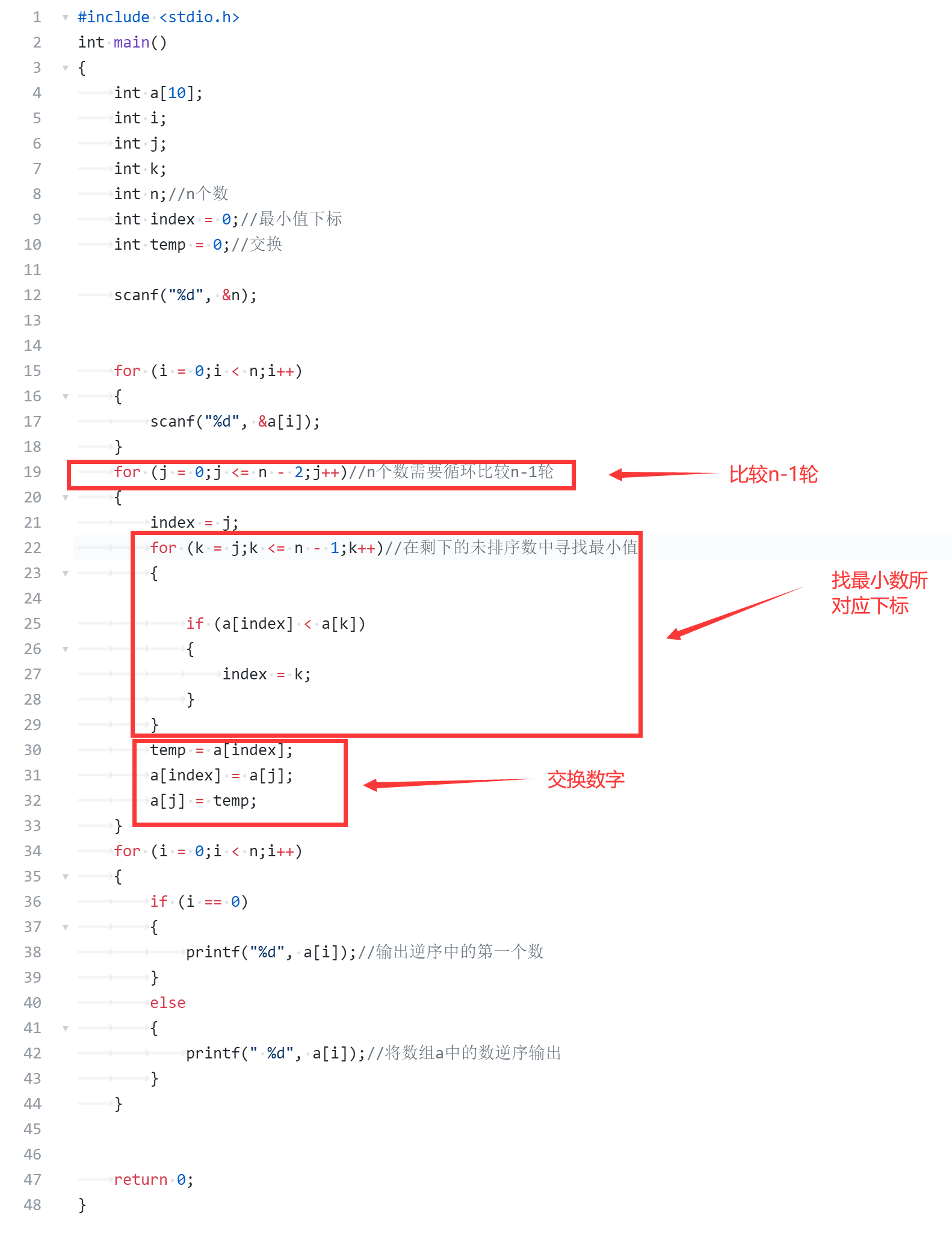#### 1.1.5.2 冒泡排序法

以从小到大排列为例：

i=0      a~a[n-1]两两比较
i=1      a~a[n-2]两两比较
i        a~a[n-i-1]两两比较
i=n-2    a~a两两比较


for i=0 to n-2 do
for j=0 to n-i-1 do
if  a[j]大于a[j+1]    //相邻的数两两比较大小
交换a[j]与a[j+1]
end if
end for
end for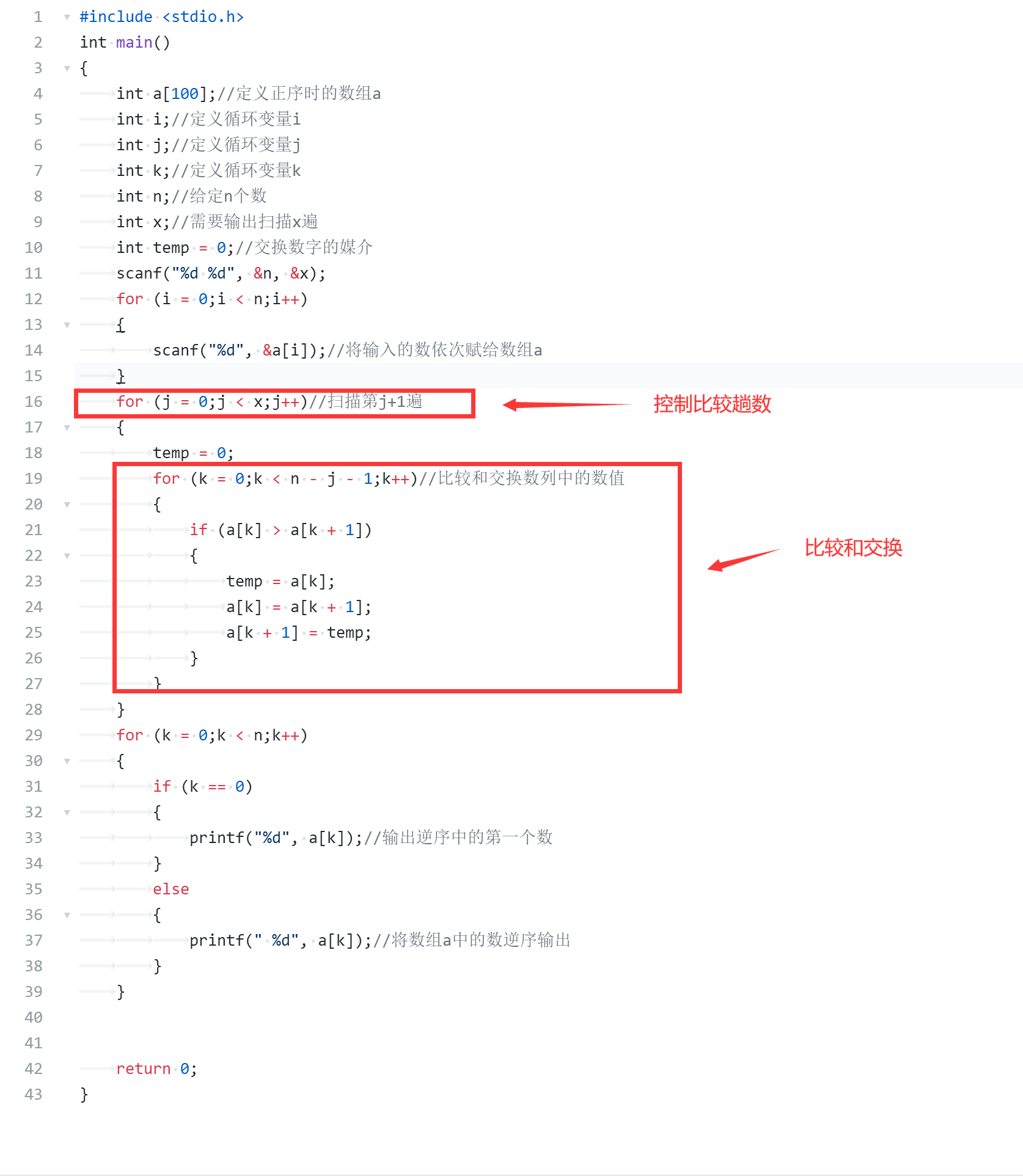### 1.1.6 数组枚举

#### 1.1.6.1 日期计算

定义一个两行十三列的二维数组，进行数组初始化，将闰年和非闰年每月的天数赋给数组，判断是否闰年，计算天数。


int k, leap;
int tap =
{
{0,31,28,31,30,31,30,31,31,30,31,30,31},//初始化，非闰年
{0,31,29,31,30,31,30,31,31,30,31,30,31}//初始化，闰年
};

leap = (year % 4 == 0 && year % 100 != 0 || year % 400 == 0)//判断是否闰年

for (k = 1;k < month;k++)//计算天数
{
day = day + tap[leap][k];
}
return day;


### 1.1.7 哈希数组

#### 1.1.7.1 删除重复字符

定义一个哈希数组hush，初始化为0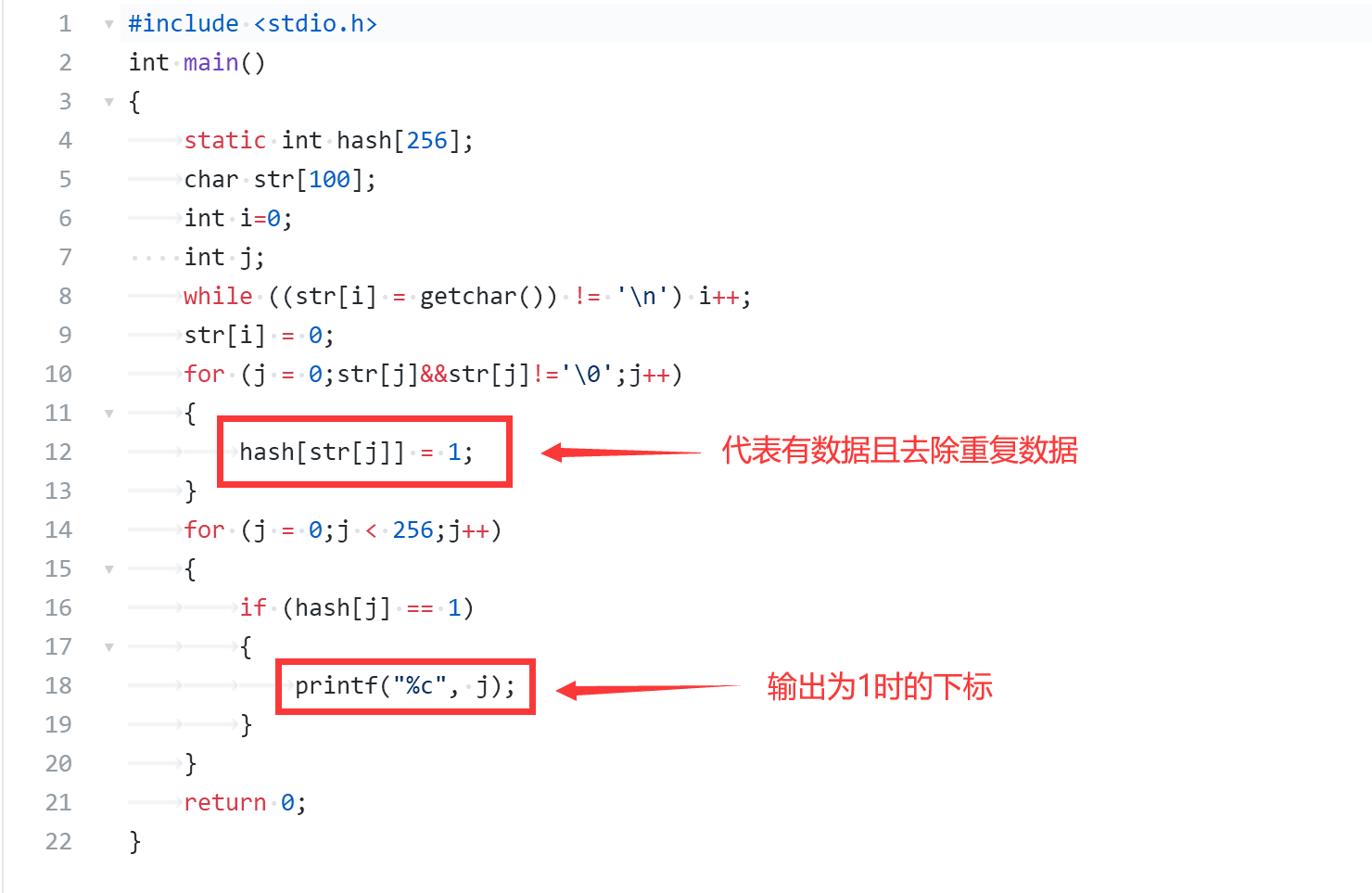# 2. PTA实验作业

## 2.1 数组循环左移

### 2.1.1 伪代码

定义一个数组a;

if n大于等于m
loc=n-m;
else
loc= n - m % n;
for i=0 to n do
m = loc % n;
输入一个数，赋值给a[m];
loc++;
end for



### 2.1.2 代码截图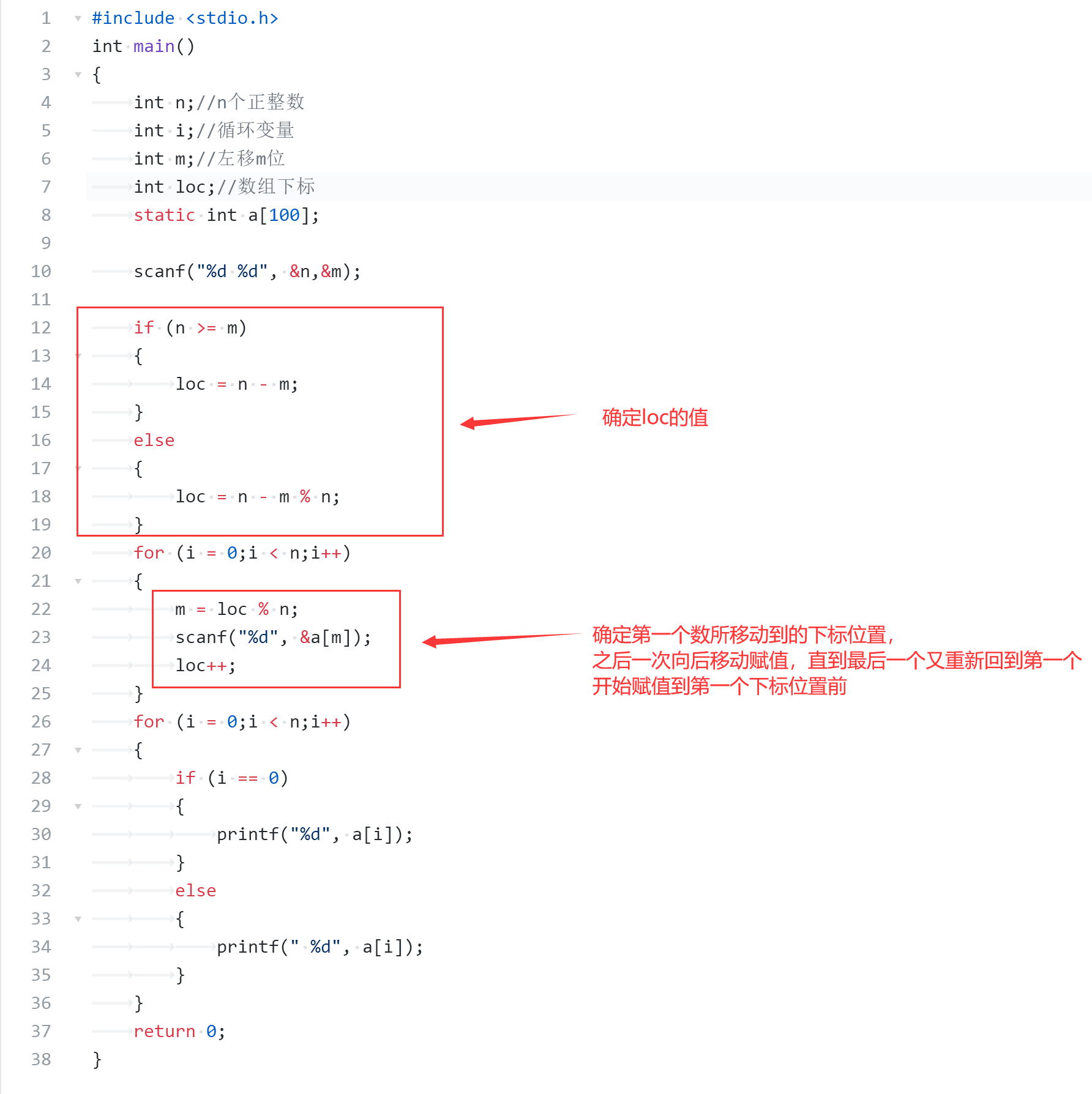### 2.1.3 代码对比分析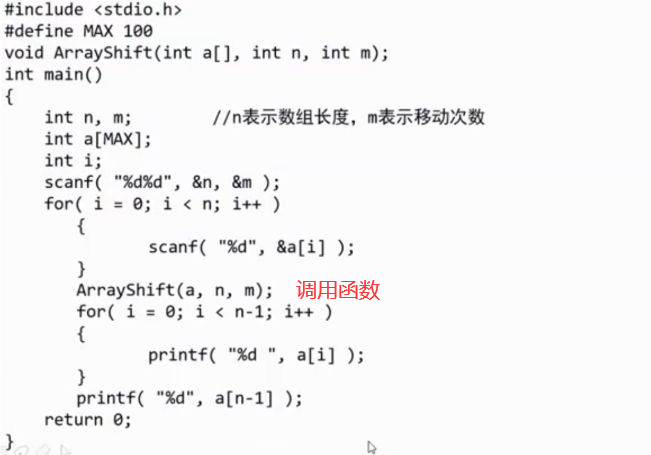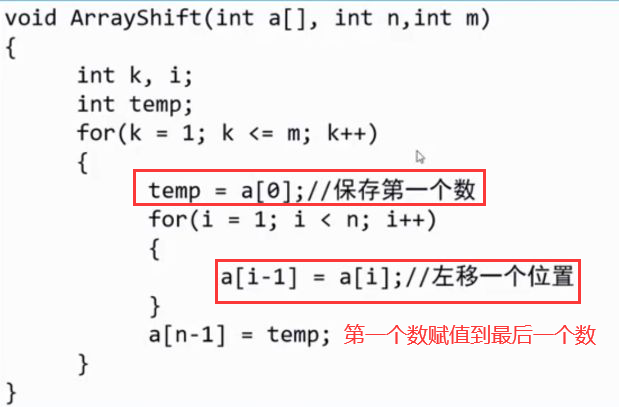• 我是根据采用寻找数据之间的关系来找到与移动的数字有关系的下标loc，然后根据取余等操作使赋值从移动到的位置开始，到最后，再到零下标开始赋值
• 她是将数值统一向前移动，然后把第一位赋值到最后一位
• 她的代码比较好理解，而且简洁明了，使用数组移动完成；而我的代码比较繁杂，需要多次scanf，而且关键下标loc需要大量数据推测找出
• 我的代码最困难的是寻找关键的下标loc，使其可以令移动到后面的数值赋值到后面，而且后面的数值下标取余到前面

## 2.2 找鞍点

### 2.2.1 伪代码

输入二维数组数据
for i=0 to n do
for j=0 to n do
for k=j+1 to n do
比较第i行中数据大小，找到最大的数的列下标maxindex；
end for
end for
同理，比较第maxindex列中数据大小，找到最小的数的行下标minindex；
if minindex 与 此时的i相等   找到鞍点flag=1；
end for
if flag=1  输出鞍点的行下标，列下标
if flag=0  输出NONE


### 2.2.2 代码截图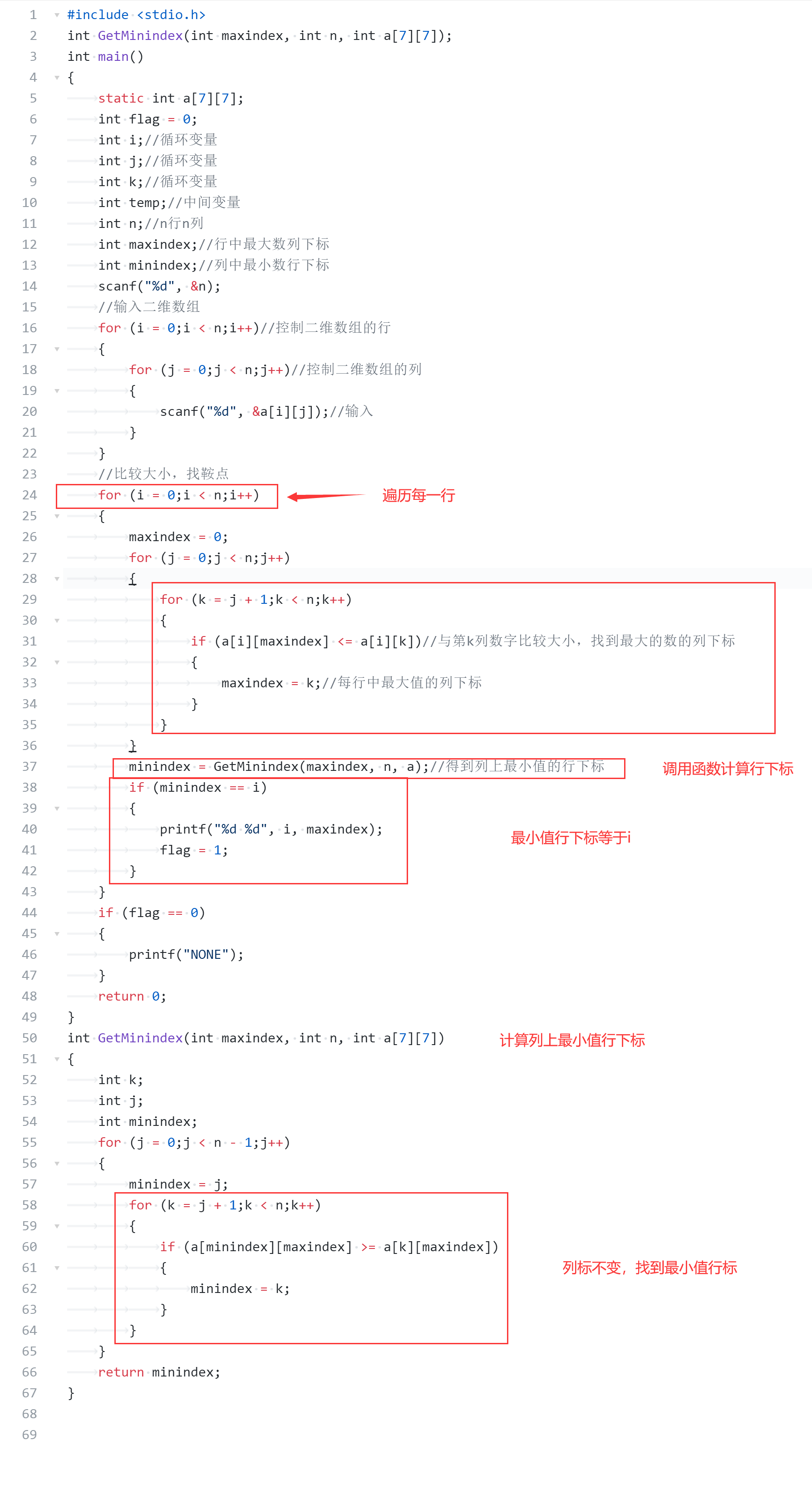### 2.2.3 两种代码对比分析

两种做法是一致的，超星视频中把整个寻找过程放在一个函数中，我把寻找行下标的过程放在一个函数中调用到寻找列下标之后。

* 使用break，提前退出循环，使程序更加有效率


• 使用break可提前退出循环，提高程序效率
• 灵活表示二维数组元素
if (a[i][j] >= a[i][maxindex] //同一行，行标相同
if (a[j][maxindex] < a[i][naxindex])  //同一列，列标相同

• 二维数组：两层循环操作

## 2.3 切分表达式

### 2.3.1 代码思路

需要思考以下几种情况
* 数字与数字一起时，不换行
* 小数点与前面的数字一起输出，不换行
* 数字后是运算符时，换行
* 当加减运算符在前面没有字符时或前面是'('时，为正负号，与数字一起输出不换行
* 其余情况都换行


### 2.3.2 伪代码

for(i=0; str[i]!='\0';i++)
if str[i] 是数字或小数点
输出str[i]
if str[i+1]不是数字，不是小数点      //数字后为运算符
输出换行符
else
if str[i]为'+'或'-'
if str[i-1]为'('或i=0      //加号或者减号前面出现（ 或者什么都没有
输出str[i]      //为正负号
else  输出str[i]和换行符      //为加减号
else 输出str[i]和换行符
end for



### 2.3.3 代码截图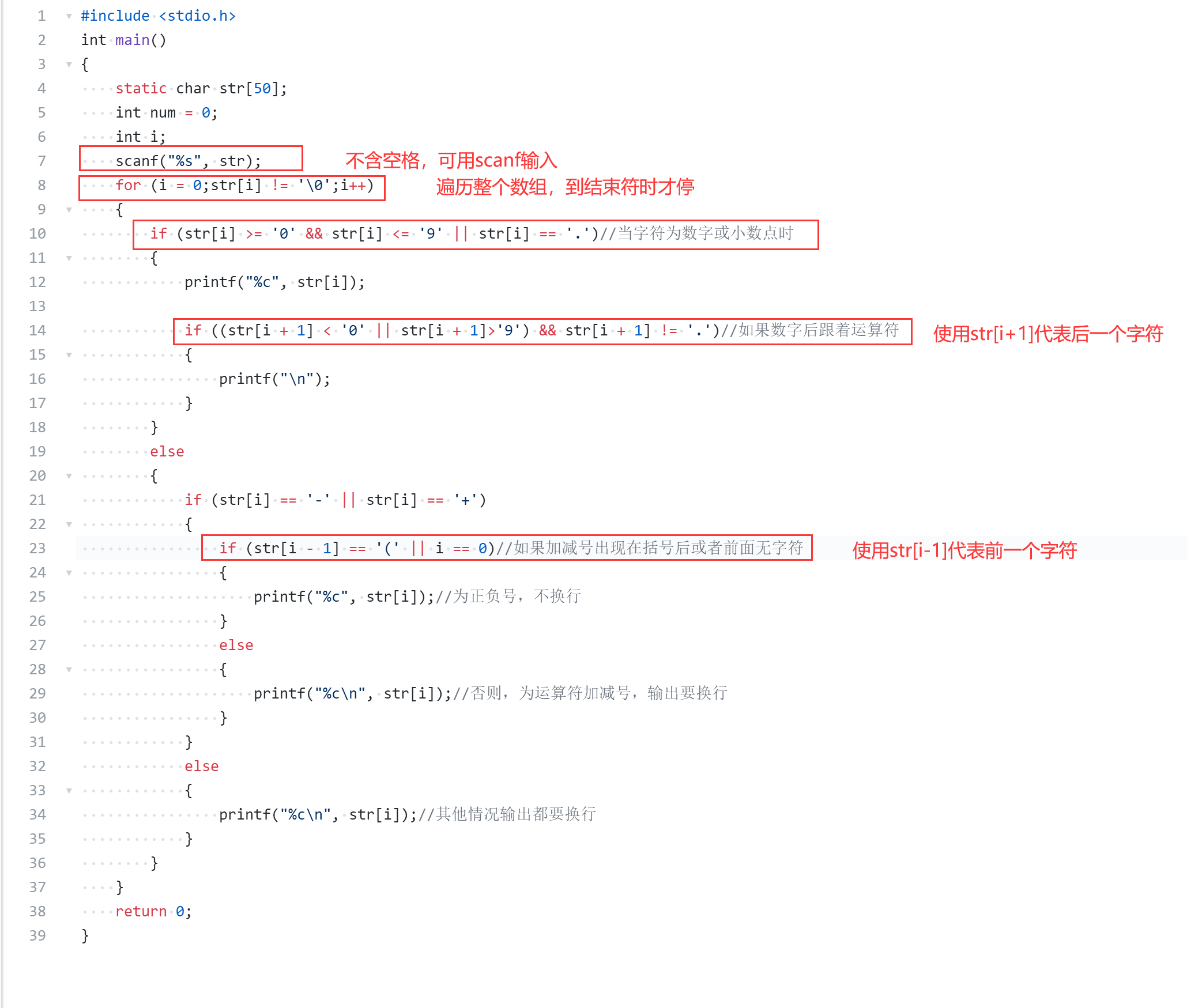### 2.3.4 两种代码对比分析

做法总题思路差不多，我的代码是先考虑特殊情况，再一般情况直接输出，超星视频里是先考虑一般情况，再考虑特殊情况

* 使用函数分装结构，使代码更加简洁明了
* 使用continue提前结束本次循环，提高程序效率


• 字符数组输入方式有三种，使用情况各不相同
• 字符数组循环停止条件可用 str[i]!='\0' 来表示
• 字符数组可通过控制下标来表示想表示的字符，如 str[i+1],str[i-1]
• 可使用continue提前结束本次循环，提高程序效率；
posted @ 2020-12-13 22:26  栀夏~  阅读(694)  评论(0编辑  收藏  举报